Thermochemistry Reaction Temperature and Pressure Quiz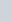Question 6 of 20 >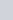8Consider the reaction.Using the standard enthalpies of formation, what is the standard enthalpy of reaction?

2 Fe203 4 3 02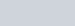= +824.2 kJ CO(g) + H20(g) —s C02(g) + H2(g)

The formation of 86.0 g of Fe results inmol

O the absorption of 1270 kJ of heat.

O the release of 1270 kJ of heat.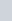Question 19 of 20 > o the absorption of 17700 kJ of hea O the release of 317 kJ of heat. O the release of 17700 kJ of heat.

o the absorption of 317 kJ of heat.Question 16 of 20 >energy change =Calculate

Calculate

Allsolution(KC1) = 983.5 J/mol.an

change,

mass =

Question 13 of 20 >

 Substance aluminum Specific heat0.897 graphite 0.709 rhodium 0.243 titanium 0.523 tungsten 0.132 zmc 0.388 water 4.184A hot 114.1 g lump of an unknown substance initially at 162.6 oc is placed in 35.0 mL of water initially at 25.0 oc and the system is allowed to reach thermal equilibrium. The final temperature of the system is 37.8 oc.

Using this information and the specific heat values for several metals in the table, identify the unknown substance.

Assume no heat is lost to the surroundings.

O tungsten O aluminum

graphite rhodium

Question 17 of 20 >

kJ

7

 General Chemistry 4th Edition 00University Science Books

presented by Macmillan Learning

Sulfur dioxide, S02 (g), can react with oxygen to produce sulfur trioxide, S03 (g), by the reaction

2 S02(g) + 02(g) 2 sq (g)

The standard enthalpies of formation for S02 (g) and S03 (g) are

AHF [S02(g)] = -296.8 kJ/m01

AHF = -395.7 kJ/m01

Calculate the amount Of energy in the form Of heat that is produced when a volume Of 1.72 L Of S02 (g) is converted to 1.72 L Of S03(g) according to this process at a constant pressure and temperature Of 1.00 atm and 25,0 oc. Assume ideal gas behavior.

energy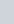Question 14 of 20 >An irregular lump of an unknown metal has a measured density of 3.57 g/mL. The metal is heated to a temperature of 163 oc and placed in a graduated cylinder filled with 25.0 mL of water at 25.0 QC. After the system has reached thermal equilibrium, the volume in the cylinder is read at 32.0 mL, and the temperature is recorded as 43.7 oc. What is the specific heat of the unknown metal sample? Assume no heat is lost to the surroundings.

Question 18 of 20 >The combustion of 1.516 g of carbon disulfide, CS2(l), in a bomb calorimeter with a heat capacity of 4.40 k.J/0C results in an increase in the temperature of the calorimeter and its contents from 22.66 oc to 31.44 cc. CWhat is the internal energy change, AU, for the combustion of 1.516 g of carbon disulfide?

At-I =kJ

Calculate the enthalpy of combustion, AHc, for the of carbon disulfide in kilojoules per mole.

Allc –kJ/mol

20A 0.981 mol sample of C02 (g) initially at 298 K and 1.00 atm is held at constant volume while enough heat is applied to raise the temperature of the gas by 12.1 K.Assuming ideal gas behavior, calculate the amount of heat (q) in joules required to affect this temperature change and the total change in internal energy, AU. Note that some books use AE as the symbol for internal energy instead of AU.IYpe of gas Molar heat capacity at constant volume atoms linear molecules nonlinear molecules 3R

where R is the ideal gas constantQuestion

kJ/molQuestion

10

of

20

>12

 The temperature of an object increases by 28.5 oc when it absorbs 3657 J of heat. CalculateThe mass of the object is 333 g. Use the table of specific heat values to identify the compositionThe Object is composed Of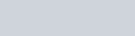Irono aluminum.gold.

the heat capacity of the object.

J/OC

of the object.Question 5 of 20 >

Which of the substances have a standard enthalpy of formation, Al-IF, equal to zero?C(diamond)

Question 15 of 20 >910 J/(kg.K), and 4190 J/(kg.K).A

295

g

silver

figure

of

liquid

water

at

22.9

when

they

reach

thermal

final temperature:Question 11 of20 >Substance Specific heat J/(g • 0C) lead 0.128 silver 0.235 copper 0.385 iron 0.449 aluminum 0.903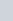Question 5 of 20 >The enthalpy changes, AH, for three reactions are given.

H2(g) + 102(g) —i H20(1) AH = -286 kJ/m01 ca(s) + 2H+(aq) —i Ca2+(aq) + H2(g) AH = —544 kJ/mol

CaO(s) + 2H+(aq) —i Ca2+(aq) + H20(1) AH = -193 kJ/m01

Using Hess’s law, calculate the heat of formation for CaO(s) using the reaction shown.

ca(s) + 102(g) —i CaO(s)

AH =kJ/mol